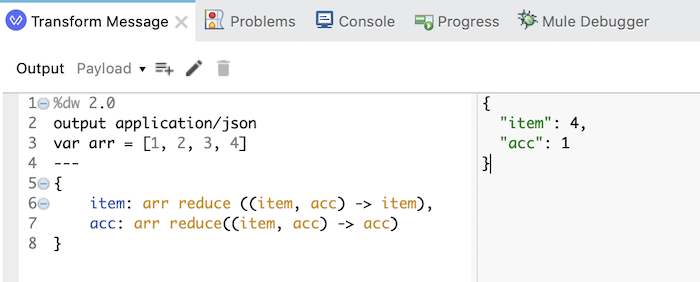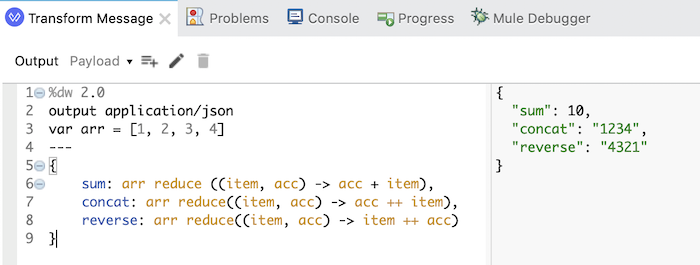# Using reduce() function in DataWeave

The reduce() function in DataWeave 1 and 2 is a function that helps us able to manipulate the elements of an array and output the type of data that we want.

To do this, the parameters of the reduce() function include the item in the array we are going to manipulate and the second parameter accumulator will store and define the type of data we want to return. The structure of the reduce() function is written in a Lambda Expression style from Java 8 as follows:

In which, <input> is the array that we will manipulate. The reduce() function will loop through all the items of the array, and for each item, it will perform the operation defined in the body of the Lambda Expression. The value of the acc parameter, if we don’t define an initial value for it, its value will start from the value of the first element of the array and we can define the return data type using this acc parameter, for example as follows:

for Object object,

for array and

for String.

For example, I have the following array:

If we do nothing:

then the last value of the item parameter will be the value of the last element in the array, and the last value of the acc parameter will be the value of the first element in the array.If you want to sum, concatenate, reverse the string, you can write the code as follows:

Result:The definition of the operation before or after the acc parameter plus defining the output in this parameter will help us get the desired result, DataWeave will rely on these definitions to return results.

By default, the value of the acc parameter will be the value of the first element in the array, but if you set it to the initial value, for example:

then the initial value of the parameter acc will be this value.

As the above example, I assigned the parameter acc an initial value of 2, so when calculating the sum of the elements of the array above, the final value will be is 12:If you have an object similar to the following:

and you want the output to be an array of student names, you can code the following:

Here, we are defining the return data type for the acc parameter as an array, so the output will see the following result:You can also use the \$ character to replace the array’s item parameter and \$\$ instead of the acc parameter when using the reduce() function, similar to the pluck() function in DataWeave.

We can write code using \$ and \$\$ as follows:

The result is still the same:## 2 thoughts on “Using reduce() function in DataWeave”

1.To get the number of elements of this array, and the first value of the acc parameter, you can code the following:

%dw 2.0

output application/json
var arr = [1, 2, 3, 4]

{
item: arr reduce ((item, acc) -> item),
acc: arr reduce((item, acc) -> acc)
}

This seems incorrect for me , because item always produces last value not the number of elements

1.